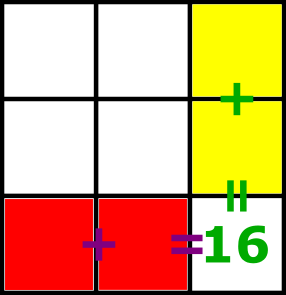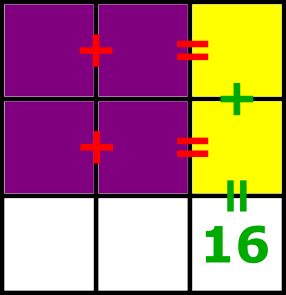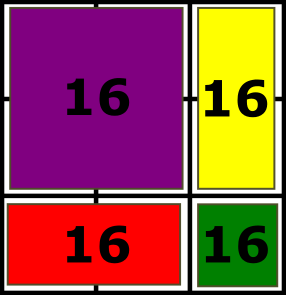#### You may also like### Picture Story

Can you see how this picture illustrates the formula for the sum of the first six cube numbers?### There and Back

Brian swims at twice the speed that a river is flowing, downstream from one moored boat to another and back again, taking 12 minutes altogether. How long would it have taken him in still water?In this problem we are faced with an apparently easy area problem, but it has gone horribly wrong! What happened?

# Table Total

##### Age 14 to 16 ShortChallenge Level

Adding up all of the numbers and symbols in the table
Total =+ 4 + (+ 4) + 8 ++ (+ 8) + (+ 8) + (+ 4) + 16

= 3$\times$+ 3$\times$+ 52

Bottom row (or last column):
(+ 8) + (+ 4) = 16, so++ 12 = 16, so+= 4

Then, multiplying by 3,
3$\times$+ 3$\times$= 12

So Total = 12 + 52 = 64

Using the rules in the table to find the totalTotal = 16$\times$4 = 64